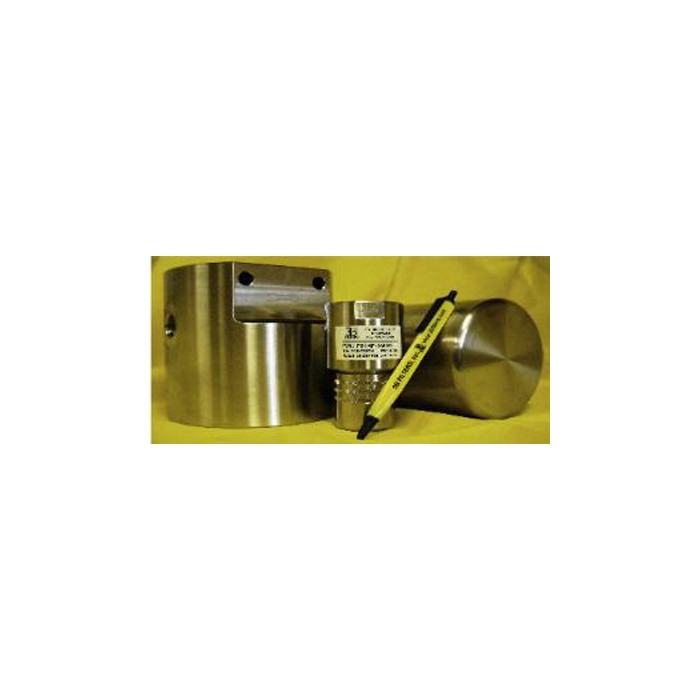Sold3B Filters

# 8100 Series Filter

8100 Series
Mini Tee-Type Filters (2.0” x 3.5”)
Up To 10,000 PSI Operating

Housing Material

• G = 316SS
• P = 17-4PH

Operating

• G = 5,000 psi
• P = 10,000psi

Proof

• G = 7,500 psi
• P = 15,000psi

Burst

• G = 20,000 psi
• P = 40,000psi

Weight

• G = 2.5Ibs
• P = 2.50Ibs

Port Size

• G = ¼ NPT
• P = ¼ NPT